#### Introducing nature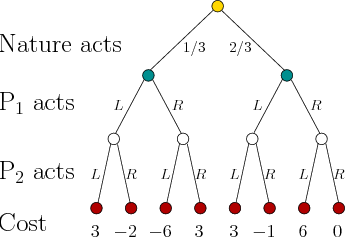A nature player can easily be introduced into a game. Suppose, for example, that nature is introduced into a zero-sum game. In this case, there are three players: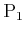,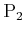, and nature. Figure 10.19 shows a game tree for a single-stage, zero-sum game that involves nature. It is assumed that all three players act at the same time, which fits the stage-by-stage model. Many other information models are possible. Suppose that probabilistic uncertainty is used to model nature, and it is known that nature chooses the left branch with probability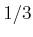and the right branch with probability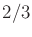. Depending on the branch chosen by nature, it appears thatandwill play a specific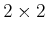matrix game. With probability, the cost matrix will be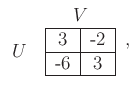(10.116)

and with probabilityit will be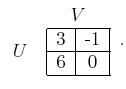(10.117)

Unfortunately,anddo not know which matrix game they are actually playing. The regret can be eliminated in the expected sense, if the game is played over many independent trials. Let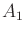and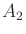denote (10.117) and (10.118), respectively. Define a new cost matrix as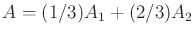(a scalar multiplied by a matrix scales every value of the matrix). The resulting matrix is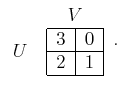(10.118)

This matrix game has a deterministic saddle point in whichchooses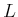(row 2) andchooses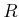(column 1), which yields a cost of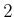. This means that they can play a deterministic strategy to obtain an expected cost of, if the game play is averaged over many independent trials. If this matrix did not admit a deterministic saddle point, then a randomized strategy would be needed. It is interesting to note that randomization is not needed for this example, even thoughandeach play against both nature and an intelligent adversary.

Several other variations are possible. If nature is modeled nondeterministically, then a matrix of worst-case regrets can be formed to determine whether it is possible to eliminate regret. A sequential version of games such as the one in Figure 10.19 can be considered. In each stage, there are three substages in which nature,, andall act. The bottom-up approach from Section 10.5.1 can be applied to decompose the tree into many single-stage games. Their costs can be propagated upward to the root in the same way to obtain an equilibrium solution.

Formulation 10.4 can be easily extended to include nature in games over state spaces. For each, a nature action set is defined as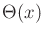. The state transition equation is defined as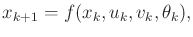(10.119)

which means that the next state depends on all three player actions, in addition to the current state. The value-iteration method can be extended to solve problems of this type by properly considering the effect of nature in the dynamic programming equations. In the probabilistic case, for example, an expectation over nature is needed in every iteration. The resulting sequential game is often referred to as a Markov game .

Steven M LaValle 2020-08-14